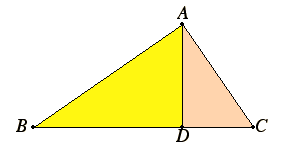Proposition 8

If in a right-angled triangle a perpendicular is drawn from the right angle to the base, then the triangles adjoining the perpendicular are similar both to the whole and to one another.

Let ABC be a right-angled triangle having the angle BAC right, and let AD be drawn from A perpendicular to BC.

I say that each of the triangles DBA and DAC is similar to the whole ABC, and, further, they are similar to one another.I.32

Since the angle BAC equals the angle BDA, for each is right, and the angle at B is common to the two triangles ABC and DBA, therefore the remaining angle ACB equals the remaining angle DAB. Therefore the triangle ABC is equiangular with the triangle DBA.

VI.4

Therefore BC, which is opposite the right angle in the triangle ABC, is to BA, which is opposite the right angle in the triangle DBA, as AB, which is opposite the angle at C in the triangle ABC, is to DB, which is opposite the equal angle BAD in the triangle DBA, and also as AC is to DA, which is opposite the angle at B common to the two triangles.

Therefore the triangle ABC is both equiangular to the triangle DBA and has the sides about the equal angles proportional.

VI.Def.1

Therefore the triangle ABC is similar to the triangle DBA.

In the same manner we can prove that the triangle DAC is also similar to the triangle ABC. Therefore each of the triangles DBA and DAC is similar to the whole ABC.

I say next that the triangles DBA and DAC are also similar to one another.

I.32

Since the right angle BDA equals the right angle ADC, and moreover the angle DAB was also proved equal to the angle at C, therefore the remaining angle at B also equals the remaining angle DAC. Therefore the triangle DBA is equiangular with the triangle ADC.

Therefore BD, which is opposite the angle DAB in the triangle DBA, is to AD, which is opposite the angle at C in the triangle DAC equal to the angle DAB, as AD, itself which is opposite the angle at B in the triangle DBA, is to CD, which is opposite the angle DAC in the triangle DAC equal to the angle at B, and also as BA is to AC, these sides opposite the right angles. Therefore the triangle DBA is similar to the triangle DAC.

Therefore, if in a right-angled triangle a perpendicular is drawn from the right angle to the base, then the triangles adjoining the perpendicular are similar both to the whole and to one another.

Q.E.D.

Corollary

From this it is clear that, if in a right-angled triangle a perpendicular is drawn from the right angle to the base, then the straight line so drawn is a mean proportional between the segments of the base.

Guide

Essentially, triangles ABC and DBA are equiangular since they are right triangles with a common angle. Therefore, they are similar. Likewise, triangles ABC and DAC are similar.

Note that Euclid verbosely draws from proposition VI.4 the conclusions that equiangular triangles are similar and that triangles similar to the same triangle are similar to each other. The general proposition that figures similar to the same figure are also similar to one another is proposition VI.21. There is no reason why that proposition could not have been placed before this one.

This proposition may be used to give an alternate proof of proposition I.47. Indeed, Euclid presents such a proof in the lemma for X.33. That proof is probably older than Euclid’s as given in I.47, but Euclid’s proof has the advantage of not being dependent on Eudoxus’ theory of proportion in Book V.

This proposition and its corollary are used in propositions VI.13, VI.31, X.33, and often in Book XIII.